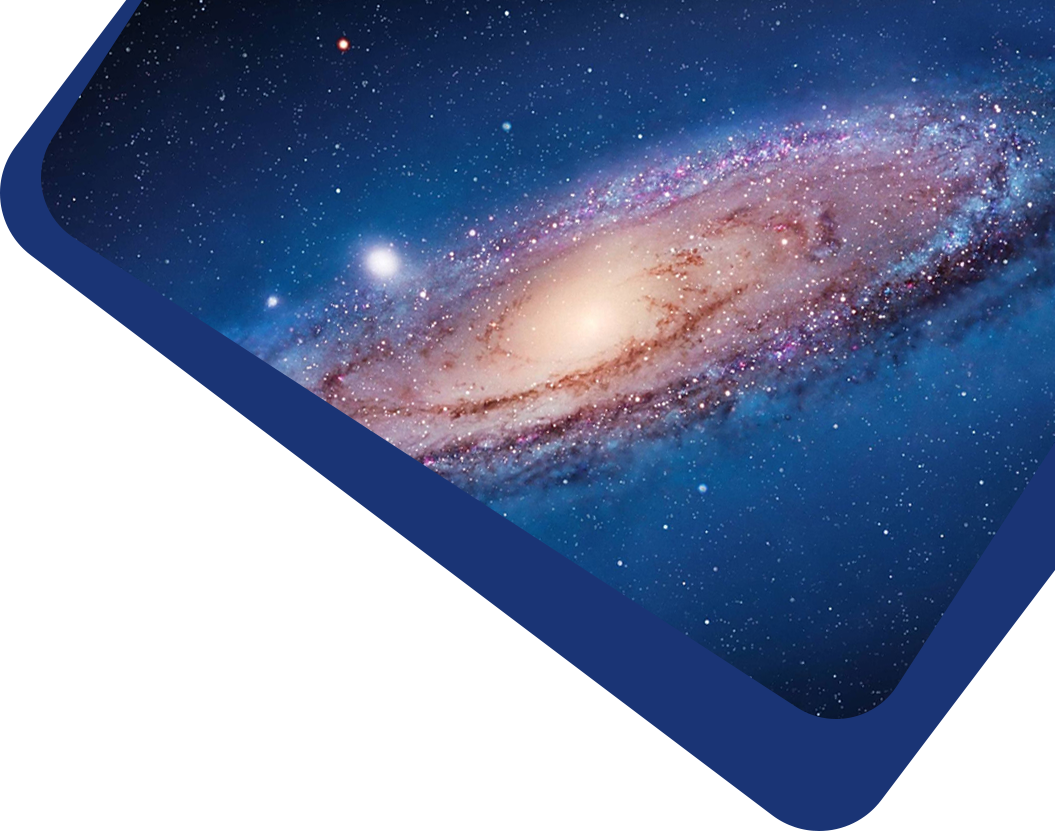Vol 18, No 4

## Statistical improvement in detection level of gravitational microlensing events from their light curves

#### Ichsan Ibrahim, Hakim L. Malasan, Chatief Kunjaya, Anton Timur Jaelani, Gerhana Puannandra Putri, Mitra Djamal

Abstract

Abstract In astronomy, the brightness of a source is typically expressed in terms of magnitude. Conventionally, the magnitude is defined by the logarithm of received flux. This relationship is known as the Pogson formula. For received flux with a small signal to noise ratio (S/N), however, the formula gives a large magnitude error. We investigate whether the use of Inverse Hyperbolic Sine function (hereafter referred to as the Asinh magnitude) in the modified formulae could allow for an alternative calculation of magnitudes for small S/N flux, and whether the new approach is better for representing the brightness of that region. We study the possibility of increasing the detection level of gravitational microlensing using 40 selected microlensing light curves from the 2013 and 2014 seasons and by using the Asinh magnitude. Photometric data of the selected events are obtained from the Optical Gravitational Lensing Experiment (OGLE). We found that utilization of the Asinh magnitude makes the events brighter compared to using the logarithmic magnitude, with an average of about 3.42 × 10−2 magnitude and an average in the difference of error between the logarithmic and the Asinh magnitude of about 2.21 × 10−2 magnitude. The microlensing events OB140847 and OB140885 are found to have the largest difference values among the selected events. Using a Gaussian fit to find the peak for OB140847 and OB140885, we conclude statistically that the Asinh magnitude gives better mean squared values of the regression and narrower residual histograms than the Pogson magnitude. Based on these results, we also attempt to propose a limit in magnitude value for which use of the Asinh magnitude is optimal with small S/N data.

Keywords

Keywords gravitational lensing: micro — methods: data analysis

Full Text
Refbacks

• There are currently no refbacks.